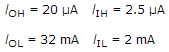# Digital Electronics - Logic Families and Their Characteristics

### Exercise :: Logic Families and Their Characteristics - General Questions

36.

Which family of devices has the characteristic of preventing saturation during operation?

 A. TTL B. MOS C. ECL D. IIL

Answer: Option C

Explanation:

No answer description available for this question. Let us discuss.

37.

How many 74LSTTL logic gates can be driven from a 74TTL gate?

 A. 10 B. 20 C. 200 D. 400

Answer: Option B

Explanation:

No answer description available for this question. Let us discuss.

38.

What is the difference between the 74HC00 series and the 74HCT00 series of CMOS logic?

 A. The HCT series is faster. B. The HCT series is slower. C. The HCT series is input and output voltage compatible with TTL. D. The HCT series is not input and output voltage compatible with TTL.

Answer: Option C

Explanation:

No answer description available for this question. Let us discuss.

39.

From the following specifications determine the fan-out for the logic family.A. HIGH state is 16, LOW state is 8 B. HIGH state is 8, LOW state is 16 C. HIGH state is 4, LOW state is 8 D. HIGH state is 8, LOW state is 4

Answer: Option B

Explanation:

No answer description available for this question. Let us discuss.

40.

Why are the maximum value of VOL and the minimum value of VOH used to determine the noise margin rather than the typical values for these parameters?

 A. These are worst-case conditions. B. These are normal conditions. C. These are best-case conditions. D. It doesn't matter what values are used.

Answer: Option A

Explanation:

No answer description available for this question. Let us discuss.

#### Current Affairs 2021

Interview Questions and Answers